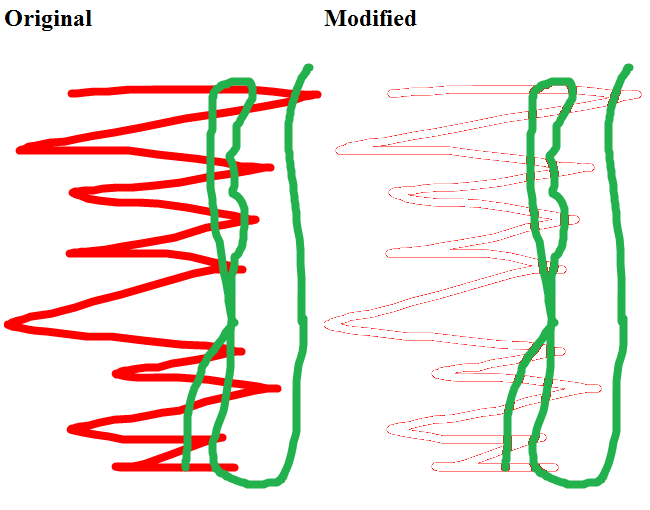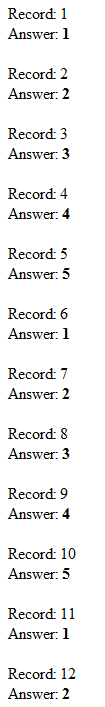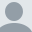# ColdFusion: Counting to a number and repeating

This post is more than 2 years old.

Ok, this is something I've done a thousand times before, but last night when I tried to get it working, it took me a few hours to get it right. I'm sure the first comment on this entry will be a comment showing how much of a bonehead I was, but darnit, I had to get this working or give up programming forever. So what's the problem? Given a set of records, I want to number them from 1 to 5 so that after 5 I go back to 1 again. Or, given 12 records, I want to see: 1,2,3,4,5,1,2,3,4,5,1,2. Simple, right?

So, I began with a simple script that I could use for testing:

`<cfset records = 12> <cfset toCount = 5>`

`<cfloop index="x" from="1" to="#records#">`

``````&lt;cfoutput&gt;
Record: #x#&lt;br/&gt;
&lt;br/&gt;
&lt;/cfoutput&gt;
``````

`</cfloop>`

Records simply represent how many iterations I'll be simulating and "toCount" represents the number I'll be counting "up" to and repeating. I then thought about the problem and came up with a formula I wrote out like this:

Take your current number, and subtract from that the whole number of sets (sets being what you are counting up).

So given 12, for example, I have 2 whole sets of 5, for a value of 10. 12-10 is 2. So here is my first take at this:

`<cfset records = 12> <cfset toCount = 5>`

`<cfloop index="x" from="1" to="#records#">`

``````&lt;cfset answer = x - (toCount * (x \ toCount))&gt;
&lt;cfoutput&gt;
Record: #x#&lt;br/&gt;
Answer: &lt;b&gt;#answer#&lt;/b&gt;&lt;br/&gt;
&lt;br/&gt;
&lt;/cfoutput&gt;
``````

`</cfloop>`

And the result:What the hey? Every time I hit a multiple of 5 I end up with 0. Ok, that makes sense I guess. So what can I do? Just reduce my current row by one. Yeah, that's it:

`<cfset records = 12> <cfset toCount = 5>`

`<cfloop index="x" from="1" to="#records#">`

``````&lt;cfset answer = x - (toCount * ((x-1) \ toCount))&gt;
&lt;cfoutput&gt;
Record: #x#&lt;br/&gt;
Answer: &lt;b&gt;#answer#&lt;/b&gt;&lt;br/&gt;
&lt;br/&gt;
&lt;/cfoutput&gt;
``````

`</cfloop>`

And the result...So - please - someone tell me there is a much simpler way of doing this?

Raymond Camden's Picture

## About Raymond Camden

Raymond is a senior developer evangelist for Adobe. He focuses on document services, JavaScript, and enterprise cat demos. If you like this article, please consider visiting my Amazon Wishlist or donating via PayPal to show your support. You can even buy me a coffee!

Lafayette, LA

### Archived CommentsComment 1 by Will B. posted on 2/22/2011 at 7:16 PM

My right-off-the-top-of-my-head thought is two counters. Inside the loop, increment the 1-to-5 counter and if GT 5 (before using), set to 1. Quickest way I can come up with.

I'm sure there's some more complex mathematical methods, if you were so inclined.

- Will B.Comment 2 by Raymond Camden posted on 2/22/2011 at 7:20 PM

One thing I should have added - I wanted to avoid any IF conditions (or trenary conditions).Comment 3 by Daniel Budde posted on 2/22/2011 at 7:29 PM

I don't know that this is any more elegant of a solution, but you could place inside your loop:

<cfif x lte toCount>
<cfset answer = x />
<cfelseif x mod toCount eq 0>
<cfset answer = toCount />
<cfelse>
<cfset answer = (x mod toCount) />
</cfif>Comment 4 by Andrea Colanicchia posted on 2/22/2011 at 7:30 PM

<cfset records = 12>
<cfset toCount = 5>

<cfloop index="x" from="1" to="#records#">

<cfset answer = ((x -1) MOD toCount) + 1>
<cfoutput>
Record: #x#<br/>
Answer: <b>#answer#</b><br/>
<br/>
</cfoutput>
</cfloop>

Not tested...Comment 5 by Daniel Budde posted on 2/22/2011 at 7:30 PM

Dag nabbit, you posted the 'no IF' while I was in the middle of writing that out.Comment 6 by Lance posted on 2/22/2011 at 7:30 PM

Don't know if it is any simpler. But her is an alternate method to computer answer.

<cfset answer = ((x-1) mod toCount) + 1>Comment 7 by Joy Anderson posted on 2/22/2011 at 7:49 PM

<cfset records = 12>
<cfset toCount = 5>
<cfset answer = 1>

<cfloop index="x" from="1" to="#records#">
<cfif answer IS toCount>
<cfset answer = 1>
<cfelse>
<cfset answer = answer + 1>
</cfif>
<cfoutput>
Record: #x#<br>
Answer: #answer#<br>
<br>
</cfoutput>
</cfloop>Comment 8 by Bob W posted on 2/22/2011 at 8:06 PM

+1 to Andrea's answer, same as what I came up with (Lance's is the same as well) - not much simpler than your answer but I think it is a bit more clear.Comment 9 by Pat Coyne posted on 2/22/2011 at 9:39 PM

Another variant on mod. Dunno if it's any clearer.

<cfset records = 12>
<cfset toCount = 5>
<cfset n=1>
<cfloop index="x" from="1" to="#records#">

<cfoutput>

Record: #n#<br/>
<br/>
<cfif #n# mod #toCount# is 0>
<cfset n=0>
</cfif>
</cfoutput>
<cfset n=#n#+1>
</cfloop>Comment 10 by Phillip Senn posted on 2/22/2011 at 11:50 PM

+1 Andrea.
Here it is in Microsoft SQL Server, where the percent sign is the MOD operator:

DECLARE @myTable TABLE(
TableID Int Identity
,Answer Int
)
INSERT INTO @myTable(Answer) VALUES(1)
INSERT INTO @myTable(Answer) VALUES(2)
INSERT INTO @myTable(Answer) VALUES(3)
INSERT INTO @myTable(Answer) VALUES(4)
INSERT INTO @myTable(Answer) VALUES(5)
INSERT INTO @myTable(Answer) VALUES(6)
INSERT INTO @myTable(Answer) VALUES(7)
INSERT INTO @myTable(Answer) VALUES(8)
INSERT INTO @myTable(Answer) VALUES(9)
INSERT INTO @myTable(Answer) VALUES(10)
INSERT INTO @myTable(Answer) VALUES(11)
INSERT INTO @myTable(Answer) VALUES(12)

SELECT *
,((Answer-1) % 5+1) AS ModAnswer
FROM @myTable
order by AnswerComment 11 by Shrainik posted on 2/23/2011 at 12:43 PM

x = 11;
for(var i=0;i<=x;i++){
output( i%5 + 1 );
}Comment 12 by Jonathan posted on 2/25/2011 at 12:28 PM

<cfset records = 12>
<cfset toCount = 5>

<cfloop index="x" from="1" to="#records#">
<cfset answer = iif(x mod toCount,x mod toCount,toCount)>
<cfoutput>
Record: #x#<br/>
Answer: <b>#answer#</b><br/>
<br/>
</cfoutput>
</cfloop>